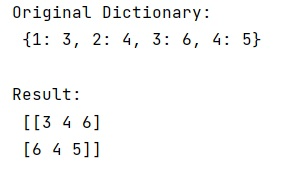# Translate every element in numpy array according to key

Learn, how to translate every element in numpy array according to key in Python?
Submitted by Pranit Sharma, on January 08, 2023

NumPy is an abbreviated form of Numerical Python. It is used for different types of scientific operations in python. Numpy is a vast library in python which is used for almost every kind of scientific or mathematical operation. It is itself an array which is a collection of various methods and functions for processing the arrays.

## NumPy Array - Translate every element according to key

Suppose we are given a dictionary and a NumPy array and we need to translate every element of a numpy.array() according to a given key.

The NumPy array contains those elements which are the keys in the dictionary and we need to replace the elements of the array with the values of each key in the dictionary.

For this purpose, we will use numpy.vectorize() method and use the get() method with the dictionary to get the values of the dictionary.

Let us understand with the help of an example,

## Python code to translate every element in numpy array according to key

```# Import numpy
import numpy as np

# Import pandas
import pandas as pd

# Creating an array
arr = np.array([1,2,-45,2,-6,3,-7,4,-2])

# Display array
print("Original array:\n",arr,"\n")

# Replacing negative values
arr[arr < 0] = 0

# Display result
print("Result:\n",arr,"\n")
```

Output: